# Archimedean solids

An Archimedean solid is a convex semi-regular solid in which the same number of regular polygons meet in the same way at every vertex, but is not a Platonic solid or prism or antiprism.

According to Pappus, Archimedes discovered 13 of them and published the result in a work which is now lost.

In the list below the number of faces, edges and vertices are listed as (F, E, V).

#### F, E, V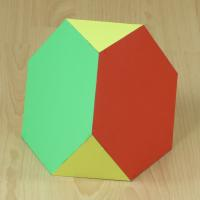Truncated tetrahedron

4 triangles, 4 hexagons
8, 18, 12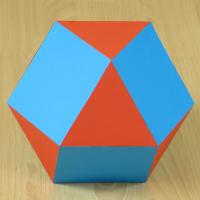Cuboctahedron

8 triangles, 6 squares
14, 24, 12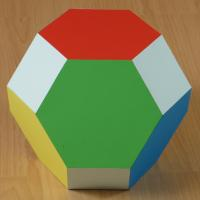Truncated octahedron

6 squares, 8 hexagons
14, 36, 24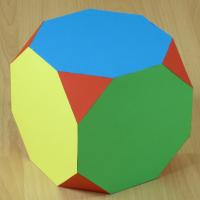Truncated cube

8 triangles, 6 octagons
14, 36, 24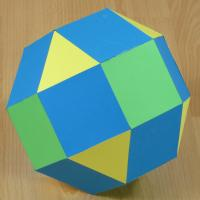Rhombicuboctahedron

8 triangles, 18 squares
26, 48, 24Truncated cuboctahedron

12 squares, 8 hexagons,
6 octagons
26, 72, 48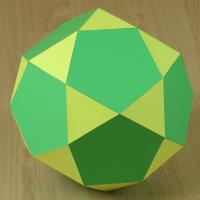Icosidodecahedron

20 triangles, 12 hexagons
32, 60, 30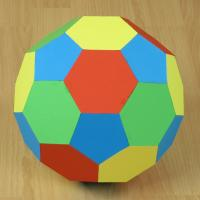Truncated icosahedron

12 pentagons, 20 hexagons
32, 90, 60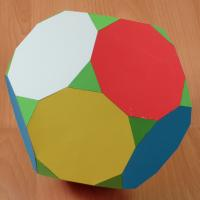Truncated dodecahedron

20 triangles, 12 decagons
32, 90, 60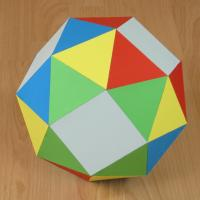Snub cube

32 triangles, 6 squares
38, 60, 24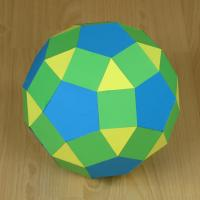Rhombicosidodecahedron

20 triangles, 30 squares,
12 pentagons
62, 120, 60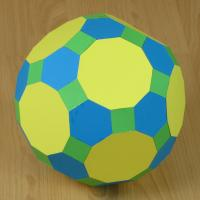Truncated icosidodecahedron

30 squares, 20 hexagons,
12 decagons
62, 180, 120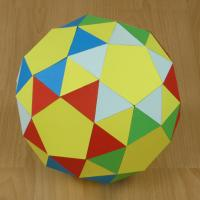Snub dodecahedron

80 triangles, 12 pentagons
92, 150, 24

Pictures are from https://www.polyhedra.net